# Thermodynamics, part 6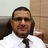- Assist.Prof of Laser Physics & Photonics en Ibb University, Yemen + Jazan University, KSA
2 de Sep de 2016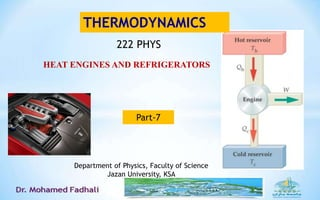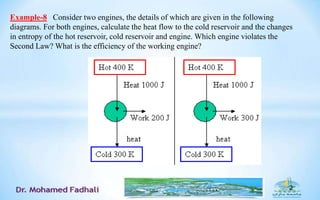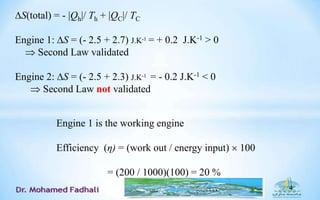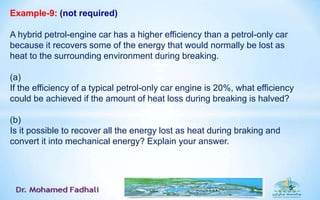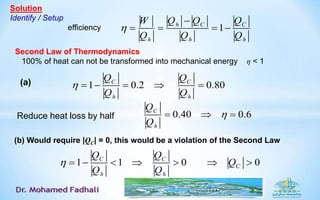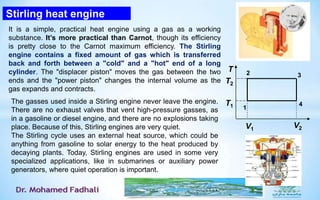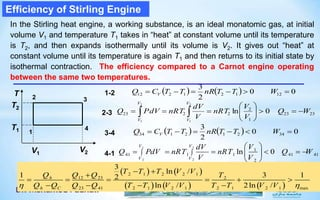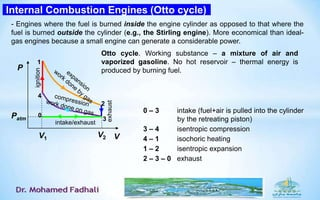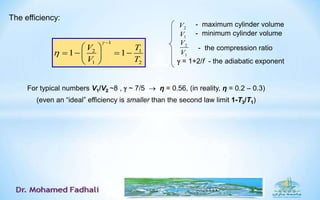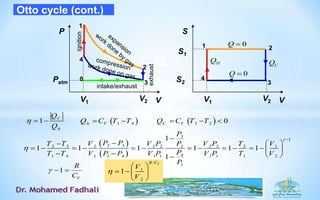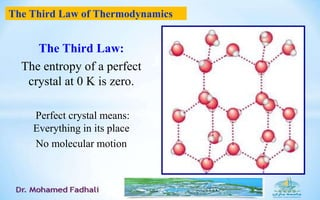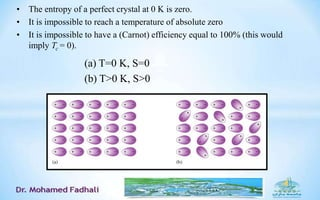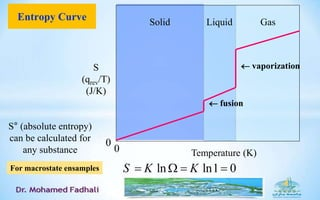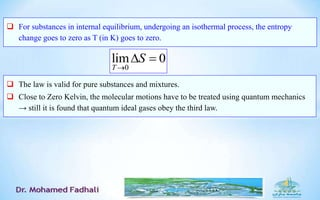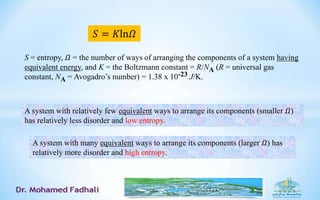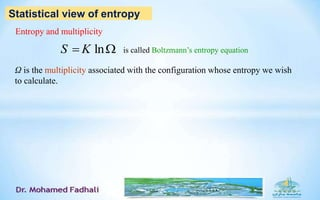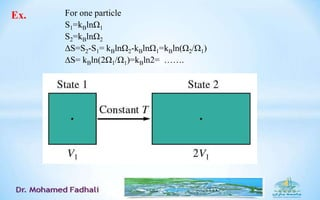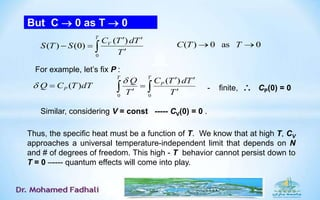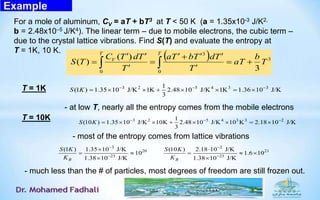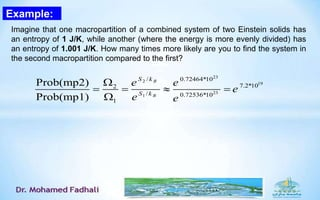1 de 23

### Thermodynamics, part 6

• 1. HEAT ENGINES AND REFRIGERATORS THERMODYNAMICS Department of Physics, Faculty of Science Jazan University, KSA Part-7 222 PHYS
• 2. 2 Example-8 Consider two engines, the details of which are given in the following diagrams. For both engines, calculate the heat flow to the cold reservoir and the changes in entropy of the hot reservoir, cold reservoir and engine. Which engine violates the Second Law? What is the efficiency of the working engine?
• 3. 3 Solution First Law: U = Q – W Engine: cyclic process U = 0  Q = W  |Qh| - |QC|  |QC | = |Qh| - W Engine 1: |QC| = 1000 - 200 = 800 J Engine 2: |QC| = 1000 - 300 = 700 J
• 4. 4 S(total) = - |Qh|/ Th + |QC|/ TC Engine 1: S = (- 2.5 + 2.7) J.K-1 = + 0.2 J.K-1 > 0  Second Law validated Engine 2: S = (- 2.5 + 2.3) J.K-1 = - 0.2 J.K-1 < 0  Second Law not validated Engine 1 is the working engine Efficiency (η) = (work out / energy input)  100 = (200 / 1000)(100) = 20 %
• 5. 5 Example-9: (not required) A hybrid petrol-engine car has a higher efficiency than a petrol-only car because it recovers some of the energy that would normally be lost as heat to the surrounding environment during breaking. (a) If the efficiency of a typical petrol-only car engine is 20%, what efficiency could be achieved if the amount of heat loss during breaking is halved? (b) Is it possible to recover all the energy lost as heat during braking and convert it into mechanical energy? Explain your answer.
• 6. Solution Identify / Setup efficiency 1h C C h h h W Q Q Q Q Q Q       Second Law of Thermodynamics 100% of heat can not be transformed into mechanical energy η < 1 (a) 1 0.2 0.80C C h h Q Q Q Q       Reduce heat loss by half 0.40 0.6C h Q Q    (b) Would require |QC| = 0, this would be a violation of the Second Law 1 1 0 0C C C h h Q Q Q Q Q        
• 7. Stirling heat engine The gasses used inside a Stirling engine never leave the engine. There are no exhaust valves that vent high-pressure gasses, as in a gasoline or diesel engine, and there are no explosions taking place. Because of this, Stirling engines are very quiet. The Stirling cycle uses an external heat source, which could be anything from gasoline to solar energy to the heat produced by decaying plants. Today, Stirling engines are used in some very specialized applications, like in submarines or auxiliary power generators, where quiet operation is important. It is a simple, practical heat engine using a gas as a working substance. It’s more practical than Carnot, though its efficiency is pretty close to the Carnot maximum efficiency. The Stirling engine contains a fixed amount of gas which is transferred back and forth between a "cold" and a "hot" end of a long cylinder. The "displacer piston" moves the gas between the two ends and the "power piston" changes the internal volume as the gas expands and contracts. T 4 1 2 3 V2V1 T1 T2
• 8. Efficiency of Stirling Engine T 4 1 2 3 V2V1 T1 T2 1-2     00 2 3 12121212  WTTnRTTCQ V 2-3 2323 1 2 2223 0ln 2 1 2 1 WQ V V nRT V dV nRTPdVQ V V V V         3-4     00 2 3 34212134  WTTnRTTCQ V 4-1 1 1 2 2 1 41 1 1 41 41 2 ln 0 V V V V VdV Q PdV nRT nRT Q W V V                        2 1 2 2 1 12 23 2 23 41 2 1 2 1 2 1 2 1 max 3 ln / 1 3 12 ln / 2ln / h h C T T T V V Q QQ T Q Q Q Q T T V V T T V V               In the Stirling heat engine, a working substance, is an ideal monatomic gas, at initial volume V1 and temperature T1 takes in “heat” at constant volume until its temperature is T2, and then expands isothermally until its volume is V2. It gives out “heat” at constant volume until its temperature is again T1 and then returns to its initial state by isothermal contraction. The efficiency compared to a Carnot engine operating between the same two temperatures.
• 9. Internal Combustion Engines (Otto cycle) Otto cycle. Working substance – a mixture of air and vaporized gasoline. No hot reservoir – thermal energy is produced by burning fuel. 0 – 3 intake (fuel+air is pulled into the cylinder by the retreating piston) 3 – 4 isentropic compression 4 – 1 isochoric heating 1 – 2 isentropic expansion 2 – 3 – 0 exhaust P V 3 4 1 2 ignition exhaust V2V1 Patm intake/exhaust 0 - Engines where the fuel is burned inside the engine cylinder as opposed to that where the fuel is burned outside the cylinder (e.g., the Stirling engine). More economical than ideal- gas engines because a small engine can generate a considerable power.
• 10. 1 2 1 1 2 1 1 V T V T             1 2 V V - the compression ratio The efficiency:  = 1+2/f - the adiabatic exponent For typical numbers V1/V2 ~8 ,  ~ 7/5  η = 0.56, (in reality, η = 0.2 – 0.3) (even an “ideal” efficiency is smaller than the second law limit 1-T3/T1) 2V - minimum cylinder volume - maximum cylinder volume 1V
• 11. Otto cycle (cont.) P V 3 4 1 2 ignition exhaust V2V1 Patm intake/exhaust 0 S V 3 4 1 2 V2V1 S2 S1 1 C h Q Q    CQHQ 0Q 0Q  1 4h VQ C T T   3 2 0C VQ C T T       3 1 2 32 3 2 2 2 2 2 2 2 1 41 4 1 1 4 1 1 1 1 1 2 1 1 1 1 1 1 1 1 1 P P PT T V V P P V P T V PT T V P P V P V P T V P                         VC R 1 / 1 2 1 VR C V V         
• 12. The Third Law: The entropy of a perfect crystal at 0 K is zero. Perfect crystal means: Everything in its place No molecular motion The Third Law of Thermodynamics
• 13. • The entropy of a perfect crystal at 0 K is zero. • It is impossible to reach a temperature of absolute zero • It is impossible to have a (Carnot) efficiency equal to 100% (this would imply Tc = 0). (a) T=0 K, S=0 (b) T>0 K, S>0
• 14. Entropy Curve Solid GasLiquid S (qrev/T) (J/K) Temperature (K) 0 0  fusion  vaporization S° (absolute entropy) can be calculated for any substance ln ln1 0S K K   For macrostate ensamples
• 15.  For substances in internal equilibrium, undergoing an isothermal process, the entropy change goes to zero as T (in K) goes to zero. 0 lim 0 T S     The law is valid for pure substances and mixtures.  Close to Zero Kelvin, the molecular motions have to be treated using quantum mechanics → still it is found that quantum ideal gases obey the third law.
• 16. S = entropy, 𝛺 = the number of ways of arranging the components of a system having equivalent energy, and K = the Boltzmann constant = R/NA (R = universal gas constant, NA = Avogadro’s number) = 1.38 x 10-23 J/K. A system with relatively few equivalent ways to arrange its components (smaller 𝛺) has relatively less disorder and low entropy. A system with many equivalent ways to arrange its components (larger 𝛺) has relatively more disorder and high entropy. 𝑆 = 𝐾ln𝛺
• 17. Statistical view of entropy Entropy and multiplicity lnS K  Ω is the multiplicity associated with the configuration whose entropy we wish to calculate. is called Boltzmann’s entropy equation
• 18. For one particle S1=kBlnΩ1 S2=kBlnΩ2 ∆S=S2-S1= kBlnΩ2-kBlnΩ1=kBln(Ω2/Ω1) ∆S= kBln(2Ω1/Ω1)=kBln2= ……. Ex.
• 19. 1 2 1 2 2 2 1 1 2 1 2 1 1 ln( ) ln( ) V V V V V V V For mole of gas S R V V For n mole of gas S nR V          Definition of entropy in term of probability
• 20. Measuring Entropy Even if we cannot calculate S, we can still measure it: For V=const and N=const : T dTTC T Q T dU dS V )(       T V T TdTC STS 0 )( )0()( By heating a cup of water (200g, CV =840 J/K) from 200C to 1000C, we increase its entropy by 373 293 (840 J/K) 202 J/K dT S T      At the same time, the multiplicity of the system is increased by 25 105.1  e holds for all reversible (quasi-static) processes (even if V is changed in the process).T Q dS   This is the “thermodynamic” definition of entropy, which Clausius introduced in 1854, long before Boltzmann gave his “statistical” definition S  kln . but Ex.
• 21. But C  0 as T  0     T V T TdTC STS 0 )( )0()( 0as0)(  TTC For example, let’s fix P : dTTCQ P )( )( 00      T P T T TdTC T Q - finite,  CP(0) = 0 Similar, considering V = const ----- CV(0) = 0 . Thus, the specific heat must be a function of T. We know that at high T, CV approaches a universal temperature-independent limit that depends on N and # of degrees of freedom. This high - T behavior cannot persist down to T = 0 –---- quantum effects will come into play.
• 22. Example For a mole of aluminum, CV = aT + bT3 at T < 50 K (a = 1.35x10-3 J/K2, b = 2.48x10–5 J/K4). The linear term – due to mobile electrons, the cubic term – due to the crystal lattice vibrations. Find S(T) and evaluate the entropy at T = 1K, 10 K.   3 0 3 0 3 )( )( T b aT T TdTbTa T TdTC TS TT V         3 2 5 4 3 31 (1 ) 1.35 10 J/K 1K 2.48 10 J/K 1K 1.36 10 J/K 3 S K           T = 1K - at low T, nearly all the entropy comes from the mobile electrons T = 10K 3 2 5 4 3 3 21 (10 ) 1.35 10 J/K 10K 2.48 10 J/K 10 K 2.18 10 J/K 3 S K            - most of the entropy comes from lattice vibrations 3 2 20 21 23 23 (1 ) 1.35 10 J/K (10 ) 2.18 10 J/K 10 1.6 10 1.38 10 J/K 1.38 10 J/KB B S K S K K K              - much less than the # of particles, most degrees of freedom are still frozen out.
• 23. Example: Imagine that one macropartition of a combined system of two Einstein solids has an entropy of 1 J/K, while another (where the energy is more evenly divided) has an entropy of 1.001 J/K. How many times more likely are you to find the system in the second macropartition compared to the first? 23 2 19 23 1 / 0.72464*10 7.2*102 / 0.72536*10 1 Prob(mp2) Prob(mp1) B B S k S k e e e e e      